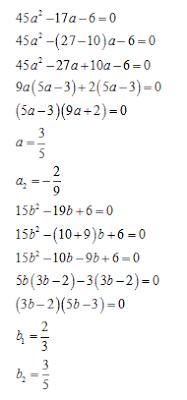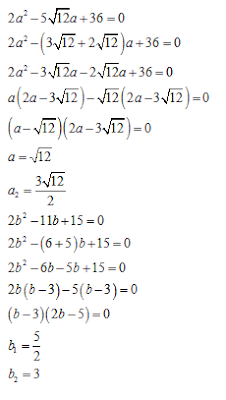# Quadratic Equations for SBI PO 2017: Part-2

1. In the following question two equations (A) and (B) given. You have to solve both:
I. 45a² - 17a -6 = 0
II. 15b² - 19b + 6 = 0

a. If a < b
b. If a < b
c. If a = b
d. If a > b
e. If a > b
2. In the following question two equations (A) and (B) given. You have to solve both:
I. 35a² - 92a + 33 = 0
II. 7b² + 39b - 18 = 0

a. If a < b
b. If a > b
c. If a = b
d. If a > b
e. If a < b

3. In the following question two equations (A) and (B) given. You have to solve both:a. If a < b
b. If a > b
c. If a > b
d. If a < b
e. If a = b

4. In the following question two equations (A) and (B) given. You have to solve both:
I. a² - 1024 = 0
II. b² - 39304 = 0

a. If a = b
b. If a > b
c. If a < b
d. If a > b
e. If a < b

5.  In the following question two equations (A) and (B) given. You have to solve both:
I. 2a² - 17a + 26 = 0
II. 2b² + 19b + 39 = 0

a. If a > b
b. If a < b
c. If a < b
d. If a = b
e. If a > b

## Solutions:

#### 1. A#### 2. B#### 3. A#### 4. E#### 5. A